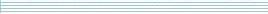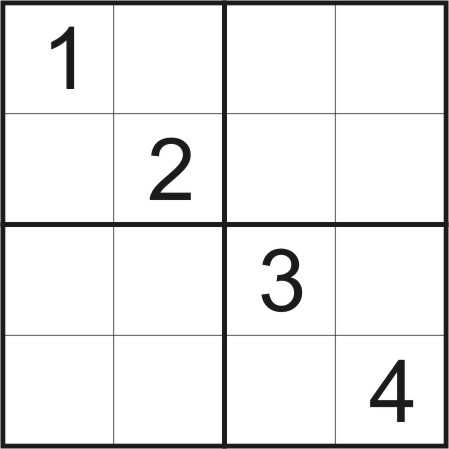| home      | people     | research     | publications    | seminars    | events     | contactCommunication Networks   | Systems Biology   | Hybrid Systems    | Machine Learning   | Dynamics & Interaction### Schools Mathematics Grand Challenge

Week three's Puzzles

Problem 7:

The seventh problem was:

Many of you have probably done SUDOKU puzzles before. Consider the SUDOKU puzzle based on the grid shown below. Each horizontal row, each vertical column and each of the four smaller grids (each containing four squares), must contain all of the numbers 1, 2, 3, 4. Usually, there is only one solution to a SUDOKU puzzle. However, in the grid below, there is more than one way of completing the puzzle. How many different completions of the grid are possible?The solution was:

Looking at the Sudoku board below, we have to put some number into the red box.The 1 and 2 can't be used, because they are already in the top-left square. So, we must use 3 or 4. Let's try 3.Once we put three in, we can first fill in the top-left square completly, and from there we can finish the problem only one way.We then go back and try putting 4 into the red box. It turns out that we can finish the Sudoku problem. Since 3 and 4 are the only possible options for the red square, and both lead us to just one solution, there are only two ways to complete the grid.

Problem 8:

The eighth problem was:

A large water tank in a country with a perfectly regular climate is exactly 48 feet high. During the rainy season, each day has exactly 12 hours of rainfall followed by 12 hours of evaporation. The water level in the tank increases at a uniform rate of 1 foot per hour during the 12 hours of rainfall, and it decreases at a uniform rate of half a foot per hour during the 12 hours of evaporation. If the tank is empty at the beginning of a 12 hour rainfall period, how many hours will it take for the water level in the tank to reach the top of the tank for the first time?

The solution was:

At the start of day 1 we have 0 feet of water in the tank. After 12 hours we have 12 feet of water and at the end of the day we have 6 feet. The pattern for day 2 is the same, but with 6 feet extra of water.

So, on day n, we finish with 6n feet of water, start with 6n-6 feet of water and the highest we get to is 6n+6 feet of water, in the middle of the day.

Let's see what the first day is when we have at least 48 feet of water. For this, 6n+6 ≥ 48, so 6n ≥ 42 or n ≥ 7. So the first day that we have at least 48 feet of water is day 7. In fact this happens in the middle of the day.

The middle of day 7 is one half day and 6 full days from the beginning. That's 24*6+12 hours, or 156 hours.

Problem 9:

The ninth problem was:

In English the most common letter is "E". We have made up a message telling you how to answer this problem and in the message the most common letter is E.

However, we have encrypted the message! For every A in the original message, we counted forward a secret number of letters in the alphabet and wrote that down. The same for B, and so on. While we are doing this counting, if we get to Z, the next letter is A.

For example, if the secret number was 2, "zebra" would become "bgdtc". This is called a Caesar Cipher after Julius Caesar who used it to talk to his generals.

In the problem below, the secret number is not 2, it is secret!

Fqjc rb nunenw crvnb bnenwcnnw?

The solution was:

The most common letter in the encrypted message is "n", so it must actually be "e". This means that we have to slide all the letters back 9 places (or, equivelently, forward 17).

When we decode the message we get:

What is eleven times seventeen?
So, the answer is 187. Some people forgot to decode the message, so we will also accept 9 and 17 as the answer. However, we'll be stricter next time!